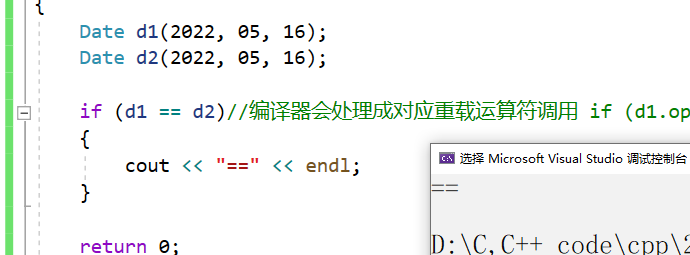## 1.运算符重载

C++为了增强代码的可读性引入了运算符重载运算符重载是具有特殊函数名的函数，也具有其返回值类型，函数名字以及参数列表，其返回值类型与参数列表与普通的函数类似。

class Date{public:  Date(int year = 1, int month = 1, int day = 1)  {    _year = year;    _month = month;    _day = day;  }private:  int _year;  int _month;  int _day;};int main(){  Date d1(2022, 05, 16);  Date d2(2022, 05, 16);    if (d1 == d2)  {     cout << "==" << endl;  }  return 0;}operator==(参数1，参数2)

bool operator==(Date d1，Date d2)

{

bool operator==(Date d1, Date d2)
{
return d1._year == d2._year
&& d1._month == d2._month
&& d1._day == d2._day;
}1、将私有变成公有。 (这种方法显然不好)

2、提供函数接口。(在类中写public的一个函数拿到私有的元素，然后类外调用这个函数间接拿到私有元素。 ---   麻烦 不建议)

3、友元。(会破坏封装，我们后面会学习到)

4、将函数放在类里。(类里可以访问，大多推荐这个)

class Date{public:  Date(int year = 1, int month = 1, int day = 1)  {    _year = year;    _month = month;    _day = day;  }//private:  int _year;  int _month;  int _day;};bool operator==(Date d1, Date d2){  return d1._year == d2._year    && d1._month == d2._month    && d1._day == d2._day;}int main(){  Date d1(2022, 05, 16);  Date d2(2022, 05, 16);  if (d1 == d2)//编译器会处理成对应重载运算符调用 if (operator==(d1, d2))  {    cout << "==" << endl;  }  return 0;}原因：这里其实不是2个参数，是3个参数，因为成员函数会包含一个隐藏的this指针。因此我们放在类中，我们就要少写一个参数。我们就应该写成这样：

class Date{public:  Date(int year = 1, int month = 1, int day = 1)  {    _year = year;    _month = month;    _day = day;  }  bool operator==(Date d)  {    return _year == d._year      && _month == d._month      && _day == d._day;  } private:  int _year;  int _month;  int _day;};int main(){  Date d1(2022, 05, 16);  Date d2(2022, 05, 16);  if (d1 == d2)//编译器会处理成对应重载运算符调用 if (d1.operator(d2))  {    cout << "==" << endl;  }  return 0;}bool operator==(const Date& d)  {    return _year == d._year      && _month == d._month      && _day == d._day;  }通过上述的例子，我们大致将运算符重载进行了了解，其中我们需要注意的是：

1、不能通过连接其他符号来创建新的操作符：比如operator@。

2、重载操作符必须有一个类类型或者枚举类型的操作数。

3、用于内置类型的操作符，其含义不能改变，例如：内置的整型+，不 能改变其含义。

4、作为类成员的重载函数时，其形参看起来比操作数数目少1成员函数的操作符有一个默认的形参this，限定为第一个形参。

5、.* 、:: 、sizeof 、?: 、. 注意以上5个运算符不能重载。这个经常在笔试选择题中出现。

（本篇完）